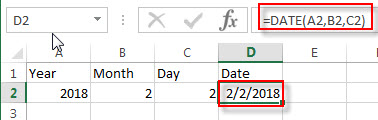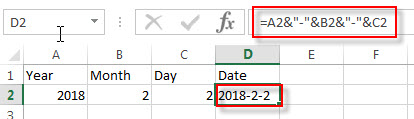# How to Combine 3 Cells to Generate One Date

This post explains that how to create a date from 3 different cells in excel. How do I combine 3 general cells to one date cell using excel formula.

Assuming that you have a list of data that contain three columns of data naming: year, month and day. You want to generate a date from combining those three columns into date cell as a standard date.

## Combine 3 Cells to Generate Date

If you want to combine 3 different cells to generate a standard data, you can create an excel formula based on the DATE function. Let’s see the below generic formula:

`=DATE(year, month, day)`

For example,

Suppose that your year value is in Cell A2, the month value is in B2, and the day value is in Cell C2. And then you can write down the following formula using DATE function:

`=DATE(A2,B2,C2)`

You can enter this formula into Cell D2, then press Enter.You can also use the concatenate operator to combine three cells to one date cell, so you can use “&” to create the below formula:

`=A1&”-“&B1&”-“&C1`

Entering this formula into cell D2, then press Enter.### Related Formulas

• Get day name from date
f you want to get the day name from a date in excel, you can use the TEXT function with a specified format code like “ddd” or “dddd”. We can try to use the below TEXT formula in excel…
• Convert date to month and year only in excel
If you want to convert the date to month and year only, you can use “yyyymm” format code within the TEXT function in excel, so you can write down the below TEXT formula:=TEXT(date,”yyyymm”)…
• Convert date to month and day only in excel
If you want to convert the date (mm/dd/yyyy) to month and day only, you can use “mm dd” format code within the TEXT function in excel, so you can write down the below TEXT formula: =TEXT(date,”mm dd”)…

### Related Functions

• Excel DATE function
The Excel DATE function returns the serial number for a date.The syntax of the DATE function is as below:= DATE (year, month, day)…